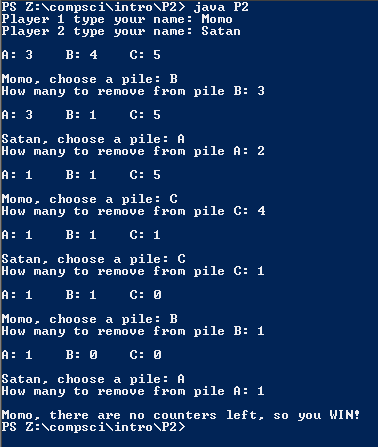# Assignemnt Project #2 Nim

## Code

```    ///Name: Morgan Kaplan
///Period: 5
///Project Name: Nim
///File Name: P2.java

import java.util.Scanner;
public class P2
{
public static void main( String[] args)
{
Scanner momo= new Scanner(System.in);

String p1, p2, player, pile;
int t, N1, end, pick, take, a, b, c;
a= 3, b= 4, c= 5, pick= 0, end= 0, t= 1, pile= "";
String p= "";

System.out.print("Player 1 type your name: ");
p1 = momo.next();

System.out.print("Player 2 type your name: ");
p2 = momo.next();

while (end == 0)
{

if  (t%2 != 0)
p= p1;

else
p= p2;

System.out.println("\nA: " + a + "\tB: " + b + "\tC: " + c + "\n");
System.out.print(p + ", choose a pile: ");
pile= momo.next();

if (pile.equals("A"))
pick= a;

else if (pile.equals("B"))
pick= b;

else if (pile.equals("C"))
pick= c;

if (pick == 0)
{
System.out.print("\nNice try, " + p + ". That pile doesn't have any. Choose again: ");
pile= momo.next();
}

else
{
System.out.print("How many to remove from pile " + pile + ": ");
take= momo.nextInt();
while (take <= 0)
{
System.out.print("\nYou must choose at least 1. How many? ");
take= momo.nextInt();
}
while ( take > pick )
{
System.out.print("\nPile " + pile + " doesn't have that many. Try again: ");
take= momo.nextInt();
}

pick= pick - take;

if (pile.equals("A"))
a= pick;

else if (pile.equals("B"))
b= pick;

else if (pile.equals("C"))
c= pick;

if ((a == 0) && (b == 0) && (c == 0 ))
{
t ++;
end = 1;
N1 = 1;
}

else
{
t ++;
N1 = 1;
}
}
}

if (t % 2 != 0)
System.out.println("\n" + p1 + ", there are no counters left, so you WIN!");

else
System.out.println("\n" + p2 + ", there are no counters left, so you WIN!");
}
} ```

### Picture of Output